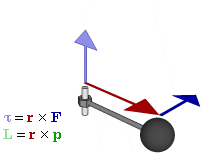## Torque with Force, Distance or Length Calculator

 I want to calculate Torque (T) Force (F) Distance or Length (D) Force (F) = N Distance or Length (D) = m Torque (T) = N-m

Torque is the tendency of a force to rotate an object about an axis. The animation below shows the Torque works.### Torque with Force, Distance or Length formula

Torque: T＝F*D

where D =Distance or Length, F =Force, T =Torque.

For example, when F is 2, Distance is 3, then Torque is 6 N-m.

Thinkcalculator.com provides you helpful and handy calculator resources.